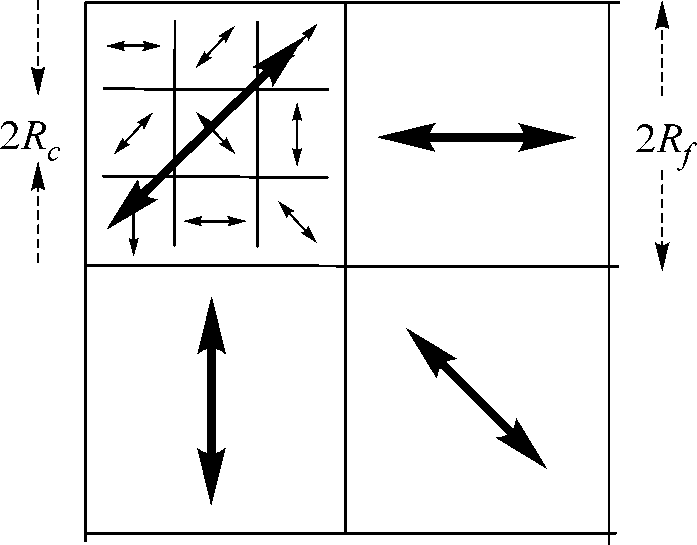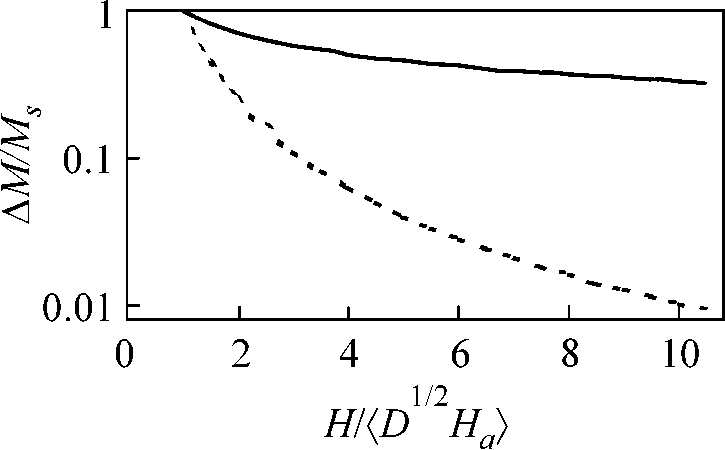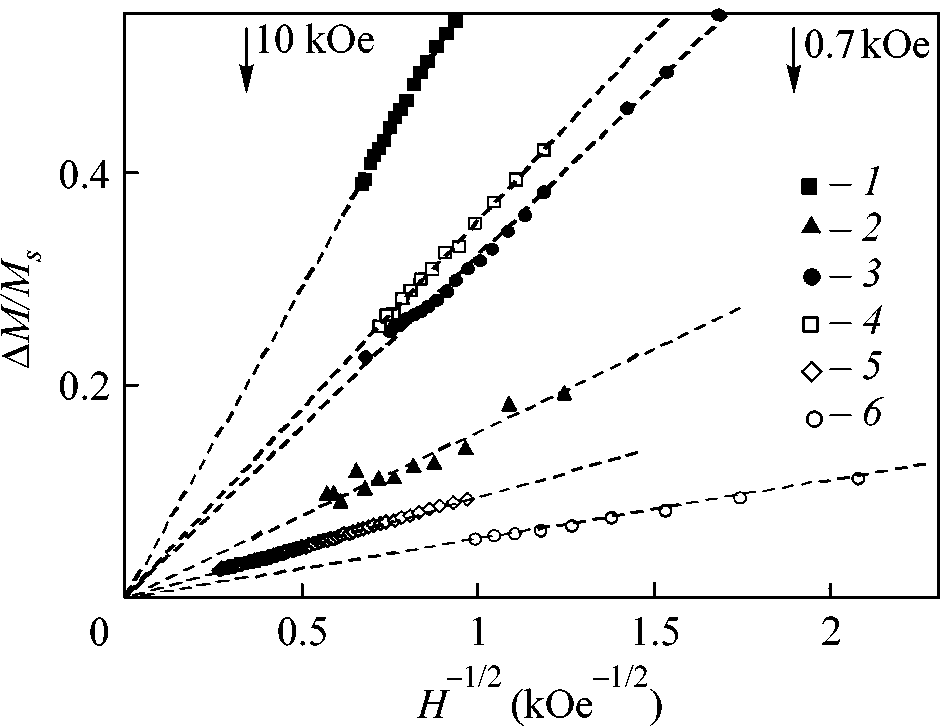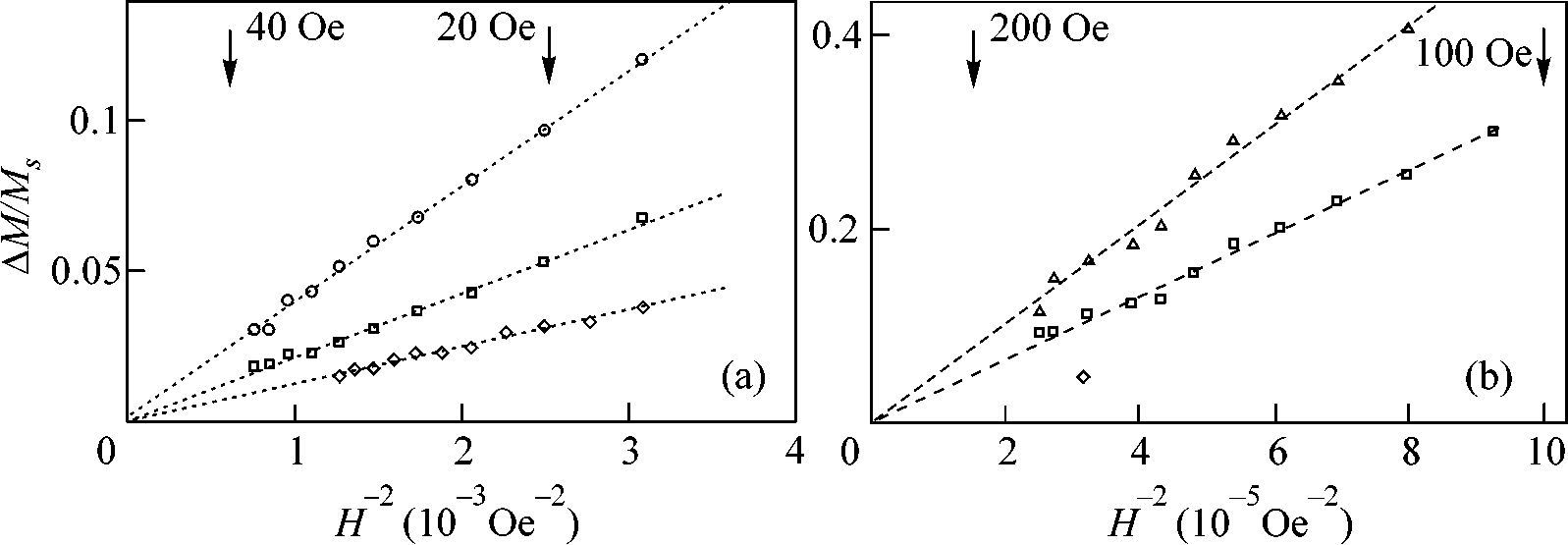## Jetplet24_00iskhakovlo

JETP Letters, Vol. 72, No. 12, 2000, pp. 603–607. Translated from Pis’ma v Zhurnal Éksperimental’noœ i Teoreticheskoœ Fiziki, Vol. 72, No. 12, 2000, pp. 872–878.
Original Russian Text Copyright 2000 by Iskhakov, Komogortsev, Moroz, Shalygina.
Characteristics of the Magnetic Microstructure
of Amorphous and Nanocrystalline Ferromagnets
with a Random Anisotropy: Theoretical Estimates
and Experiment
R. S. Iskhakov*, S. V. Komogortsev*, Zh. M. Moroz**, and E. E. Shalygina***
* Kirenskiœ Institute of Physics, Siberian Division, Russian Academy of Sciences, Akademgorodok, Krasnoyarsk, 660036 Russia ** Siberian University of Routes of Communication, Krasnoyarsk Branch, Krasnoyarsk, 660028 Russia *** Moscow State University, Vorob'evy gory, Moscow, 119899 Russia An experimental determination (both direct and indirect) of the characteristics of the magnetic microstructure,namely, the ferromagnetic correlation radius R f and the rms fluctuation of the mean anisotropy D formed for amorphous and nanocrystalline ferromagnets with a random anisotropy characterized by the quan-tities R Ha, respectively. The magnetization curves of amorphous and nanocrystalline ferromagnets are found to exhibit a dependence on H that is caused by the alignment of the magnetizations of individual magneticblocks with the field. 2000 MAIK “Nauka/Interperiodica”. PACS numbers: 75.30.Gw; 75.50.Kj; 75.60.-d 1. Amorphous and nanocrystalline ferromagnetic
anisotropy field D1/2Ha, where D is the symmetry factor alloys can be represented as an ensemble of clusters or equal to 1/15 for a uniaxial anisotropy . In the case grains of size 2Rc, which are bound together by the of large grains satisfying the inequality exchange interaction and have randomly oriented easy axes. In the approximation of a continuous medium, R > D 1/4( A/K )1/2, such a system is described by the internal energy den-sity the correlation properties of the inhomogeneous state
of the orientation of M always coincide with the corre-
lation properties of the local anisotropy fluctuations. In U = - α(∇M)2
- β(Ml)2
this case, the approximation of crystallites withoutexchange interaction between them is valid. Beginning where the magnetization M is characterized by a con- from the publications [11, 12], this approximation was used for calculating the law of magnetization approach 2A/ M2 is determined by the exchange interaction con- stant A, the parameter β = HM/M = ( DH /H)2, H > D1/2H .
a/Ms = 2K/ M is deter- mined by the local anisotropy constant K, l is the unit
For amorphous and nanocrystalline ferromagnets, the vector of the easy axis of this anisotropy, and H is the It is known that, in a ferromagnet, an orientational irregularity of the magnetic anisotropy of any origin If this inequality is satisfied, the correlation properties (crystallographic, elastic, or other) gives rise to the for- of the SMS fundamentally differ (in the fields H < Hex) mation of an inhomogeneous state of the magnetic from those of the local anisotropy: the deviations of the moment M(x) [1–10]. This state is called a stochastic
magnetization M(x) from the direction of the external
magnetic structure (SMS). The parameters of the SMS field are correlated in space and form a static wave with are determined by the relations between three charac- the characteristic wavelength R = (2A/MH)1/2. The teristic fields: the external field H, the exchange field changes occurring in the correlation properties of the ex = 2A/Ms R , and the rms fluctuation of the local SMS in the vicinity of the field Hex lead to a change in 0021-3640/00/7212-0603\$20.00 2000 MAIK “Nauka/Interperiodica”such a material can be described by an ensemble ofweakly coupled magnetic blocks (Fig. 1). The blocksize is 2R f, the mean anisotropy in the block is K = , and the unit vector n of this anisot-
ropy is randomly oriented. In the approximation of acontinuous medium, such a system can be described bythe internal energy density represented in the form U = –- βe(Mn) – HM,
where the parameter βe = 〈H a /M = 2 K /M is deter- Fig. 1. Schematic representation of a ferromagnet with a
mined by the constant 〈K〉 characterizing the mean random anisotropy. The small arrows indicate random ori- anisotropy in the magnetic block. In zero field, the entation of the local magnetic anisotropy l(x), and the large
magnetization of a magnetic block is oriented along the arrows show random orientation of the mean anisotropy of unit vector n. Therefore, in this case, the correlation
a magnetic block n(x).
properties of the irregular orientation of M(x) com-
pletely reproduce the correlation properties of the fluc-
tuations of mean anisotropy 〈K〉. This means that the
magnetization curve in low magnetic fields should be
described by dependence (3) modified as follows:
M/M = (D1/2 〈 H 〉/H)2 = (R /R )4, H > D1/2 〈 H 〉 or R < R .
Simultaneously, using the definition of the characteris-tics of the system of magnetic blocks (R in terms of the characteristics of the grain system (Rc Fig. 2. Theoretical dependences of the variance of magnetic
and D1/2Ha), Eq. (5) can be represented in the form moment on the external magnetic field in the D1/2〈H 〉 the solid curve corresponds to Eq. (11) and the dashed curveto Eq. (14).
M/M = (D1/2 〈 H 〉/H)1/2 = R /R , R < R Ӷ R .
the law of magnetization approach to saturation. For The aim of our work is the experimental study of the three-dimensional and isotropic inhomogeneities of aforementioned effects. Its significance is determined anisotropy, the following relationship was obtained by the fact that the experimental measurement of dependence (3') is a direct, rather than indirect [seeEq. (5')], proof of the existence of magnetic blocks and (D1/2H /H )2(H /H)1/2 allows one to directly measure the characteristics of the magnetic microstructure (the quantities 〈H 〉 = (D1/2H /H )2( R /R ), f) of amorphous and nanocrystalline ferromagnets.
2. Let us theoretically estimate the correlation prop-
erties of the irregular orientation of M(x). The main
For anisotropic and low-dimensional inhomogeneities, characteristic of these properties is the correlation func- the dependence on H may be different [6, 7, 13–16].
tion Km(r) or the spectral density Sm(k) related to the
correlation function through the Fourier transform:
As the magnetic field further decreases (H Ӷ Hex), the situation changes. In low magnetic fields, the mag- 〈mx
( + r)〉 = K r
netic system of amorphous and nanocrystalline ferro-magnets exhibits the well-known Imry–Ma effect .
( )m⊥* x
( + r) = S k
( )δ(k k');
This effect consists in the instability of the ferromag-netic state with respect to the randomly oriented local K r
S k
( )eikr k
magnetic anisotropy. In this case, the ferromagneticorder is characterized by the correlation length Rf = where m⊥(x) are the transverse components of the unit
Ha) [5, 10], so that the magnetic structure of vector of magnetization m(x) = M(x)/M. The magneti-CHARACTERISTICS OF THE MAGNETIC MICROSTRUCTURE zation curve is related to Km(r) and Sm(k) by the stan-
dard relationships
M/M d (H) = K r
S k
The general expression for Sm(k) through the arbitrary
spectral density S(k) of the fluctuations of local anisot-
ropy axis has the form [3, 4, 17]
  2 S k
S k
where kH = 1/RH is the wave number of exchange cor-relations. If we model the stochastic properties of theorientational irregularity of magnetic anisotropy by thesimplest correlation function Fig. 3. High-field portions of the magnetization curves
M(H): (1) Fe73.5Cu1Nb3Si13.5B9 and (2) Co80Zr10 amor- tape; Co90P10 amorphous films with t = (4) 500 and(5) 2000 Å; and (6) a Co90P10 amorphous coating with t = where kc = 1/Rc, we obtain a symmetric expression for Sm(k):
for the variance of the random deviations of 〈 m(x)〉 :
In this case, the expression for the variance d d' (H) = --------------------- --- Here, the rms fluctuation of anisotropy in a magnetic One can see that, for H ӷ Hex, Eq. (11) yields expres- block is D1/2〈H 〉 = D1/2H sion (3) for both inequalities (2) and (4) and, for H Ӷ a/(Rc/Rf)3/2 and the field Hf is ex, Eq. (11) yields expression (5).
We now consider a random function 〈 m(x)〉 that
is obtained by averaging the random function m(x) over
is reduced to Eq. (3'). Figure 2 shows dependences (11) the space interval (x – |R |
f , x + |Rf ):
and (14) as functions of magnetic field in D1/2〈H 〉 One can see that, up to H ≈ 10D1/2〈Ha , the variations of the experimental error). This means that the magnetiza- tion of amorphous and nanocrystalline ferromagnets The stochastic properties of the orientational irregu- occurs through the alignment of the mean magnetiza- larities of the mean anisotropy 〈K〉 of magnetic blocks tions of the blocks with the field [according to Eqs. (3') are determined by the rules described in . In the and (14)] and only after that the decrease in the ampli- case Rf ӷ Rc, these properties are described by the cor- tude of m⊥(x) [described by Eqs. (5), (5'), and (11)]
relation function and the spectral density of the follow- 3. Figure 3 presents the high-field portions of the
magnetization curves M(H) for films and foils of amor- phous and nanocrystalline alloys produced by different techniques (fast quenching of the melt or chemical dep- osition). The magnetization curves were obtained using where kf = 1/Rf. Substituting Eqs. (13) into Eq. (8) and vibrating-coil magnetometers with an electromagnet then into Eq. (7), we obtain the following expression for fields of up to 15 kOe and with a superconducting solenoid for fields of up to 30 kOe. One can see that, in inside the sample do not coincide with the external magnetic field strength at H ~ H s, H–1/2) coordinates, the experimental curves can be described by linear dependences (of the type y = This difficulty can be overcome with the use of an ax) determined by Eqs. (5) and (5'). This means that experimental technique that allows one to exclude the inequality (4) is valid for the amorphous and nanocrys- effect of magnetostatic mechanisms. Such a technique talline alloys under study. The experimental curves also is realized by a magneto-optic micromagnetometer suggest that the numerical value of the coefficient of  allowing the measurements of the local magnetiza- linear dependence is determined by the characteristics tion curves by using the equatorial Kerr effect δ(H) ~ of the random anisotropy, Rc and D1/2Ha. To calculate M(H) with the light spot diameter of 1 to 30 µm in mag- the latter, it is necessary to measure the magnetization netic fields of up to 200 Oe. In this technique, the exter- curve in the fields H > Hex. By recording the part of the nal magnetic field is applied along the sample surface curve described by dependence (3), one can determine normally to the plane of light incidence. Before mea- suring the local magnetization curves M(H), the system a. The revealed crossover of M(H) (the transition from ∆M ~ H–1/2 to ∆M ~ H–2) provides the possibility is tuned so as to depart from the significant magneto- static sources. For this purpose, it is necessary to con- ex. Substituting the exchange interaction constant A, which is calculated from the low-tempera- struct the distribution curves δ(L)/δs ~ M(L)/Ms at a ture thermomagnetic curves ∆M ~ (T/A)3/2, into H constant external magnetic field H (much lower than c. However, in many cases this pro- scanning the light spot over the sample surface along an gram cannot be implemented. Many amorphous and arbitrarily chosen direction. (The scan length L is cho- nanocrystalline ferromagnetic alloys are characterized sen so as to exceed the spot diameter by two to three by the values of Hex that exceed the maximal fields used orders of magnitude). Typical distribution curves can in the experimental measurements of M(H) (see, e.g., be found in . They exhibit irregular deviations of M ). Then, the grain size (or the cluster size) 2Rc can from the mean magnetization 〈M〉. As the field is be determined by direct methods such as the X-ray increased and the scanning along L is repeated, the structural analysis or transmission electron microscopy value of 〈M〉 increases and the deviations decrease, but and the values of Hex and D1/2Ha can be calculated (the the spatial scale of deviations is retained. The field Hs latter is obtained from the linear dependence ∆M ~ H–1/2 is determined as the one at which the amplitude of the deviations is of the order of experimental error. For oursamples, the following deviations were observed: For the characteristics of the magnetic microstruc- f and D1/2〈Ha ) the situation is entirely different.
For their indirect determination, it is sufficient to record the linear dependences shown in Fig. 3. The measure- ment of the coefficient of linear dependence allows one nanocrystalline tape. The local magnetization curves to calculate the rms fluctuation of the anisotropy field in M(H) were obtained from the light spot, 20–30 µm indiameter, set at the center of a soft magnetic region. It a magnetic block, D1/2〈H a Hf. The substitution of A was believed that the deviations of M(x) in this region
into Hf provides the value of Rf. For the amorphous and are caused by the scattering due to the chaotic orienta- nanocrystalline alloys presented in Fig. 3, the following tion of the axis n(x) of a magnetic block.
a and Rf were obtained: 340 Oe and Figure 4 shows the local magnetization curves M(H) 130 Å, respectively, for a Fe73.5Cu1Nb3Si13.5B9 amor- phous tape 20 µm thick; 25 Oe and 640 Å for a 73.5Cu1Nb3Si13.5B9 amorphous foil and a Co90P10 amorphous film; the curves were measured for different Co80Zr10V10 amorphous tape 30 µm thick; 100 Oe and parts of the samples. One can see that, in the (∆M/M H–2) coordinates, the experimental magnetization 20 µm thick; 120 Oe and 380 Å for a Co90P10 amor- curves are described by linear dependences of the type phous film 500 Å thick; 9 Oe and 1430 Å for a Co90P10 of Eq. (3'). The slopes of these dependences character- amorphous film 2000 Å thick; and 3 Oe and 2460 Å for ize the values of the rms fluctuation of the mean anisot- a Co90P10 amorphous coating 30 µm thick. To directly ropy field in a magnetic block, D1/2〈H f and D1/2〈Ha , it is necessary presented in Fig. 4, we obtained: D1/2〈H 〉 ≈ 3–6 Oe and to record the part of the dependence M(H) that is described by Eqs. (3') and (14) rather than by Eqs. (5), f ≈ 970–1300 Å for a Fe73.5Cu1Nb3Si13.5B9 foil and D1/2〈H 〉 ≈ 60–70 Oe and R (5'), and (11). With a vibrating-coil magnetometer, such a measurement is apparently impossible, because real film (500 Å thick). One can see that the difference amorphous and nanocrystalline alloys contain microc- between the values of D1/2〈H 〉 racks, pores, and inclusions of a second phase, giving the Co90P10 amorphous film by the direct [Eq. (3')] and rise to magnetostatic mechanisms of scattering for the indirect [Eq. (5')] methods does not exceed 50%. The magnetic moment [these mechanisms are not included greater difference between the corresponding values in Eqs. (1) and (1')]. As a result the true values of H obtained for a Fe73.5Cu1Nb3Si13.5B9 tape is no surprise.CHARACTERISTICS OF THE MAGNETIC MICROSTRUCTURE Fig. 4. Local magnetization curves M(H) measured for different parts of (a) a Fe73.5Cu1Nb3Si13.5B9 amorphous tape (t = 20 µm)
and (b) a Co90P10 amorphous film (t = 500 Å).
The point is that the local signal δ(H) ~ M(H) is 6. H. Kronmüller, IEEE Trans. Magn. 15, 1218 (1979).
obtained from a thin surface layer ~200 Å thick. There- 7. A. P. Malozemoff, IEEE Trans. Magn. 19, 1520 (1983).
fore, a coincidence between the “surface” signal M(H) 8. E. M. Chudnovsky, W. M. Saslow, and R. A. Serota, and the integral value of M(H) should be expected only Phys. Rev. B 33, 251 (1986).
for films whose thickness is comparable to the penetra- 9. W. M. Saslow, Phys. Rev. B 35, 3454 (1987).
tion depth in the magneto-optic technique. The lattercondition is fulfilled for the 500 Å-thick Co 10. G. Herzer, IEEE Trans. Magn. 26, 1397 (1990).
phous film and does not hold for the foils showing 11. N. S. Akulov, Z. Phys. 69, 278 (1931).
noticeable differences between the values of D1/2〈H 〉 12. W. F. Brown, Jr., Phys. Rev. 58, 736 (1940).
and Rf obtained for the bulk of the samples and for the 13. E. M. Chudnovsky, J. Magn. Magn. Mater. 40, 21
14. A. G. Chernykh, P. P. D’yachuk, and V. B. Kruglov, Izv.
V.P. Ovcharov for providing the films and foils and to Akad. Nauk SSSR, Ser. Fiz. 53, 622 (1989).
V.A. Ignatchenko for useful discussions and interest in 15. V. A. Ignatchenko and R. S. Iskhakov, Fiz. Met. Metall- 16. R. S. Iskhakov, S. V. Komogortsev, A. D. Balaev, et al., Pis’ma Zh. Éksp. Teor. Fiz. 72, 440 (2000) [JETP Lett.
72, 304 (2000)].
1. H. Hoffman, IEEE Trans. Magn. 2, 566 (1966).
17. Y. Imry and S.-K. Ma, Phys. Rev. Lett. 35, 1399 (1975).
2. K. J. Harte, J. Appl. Phys. 38, 1503 (1968).
18. S. M. Rytov, Introduction to Statistical Radiophysics 3. V. A. Ignatchenko, Zh. Éksp. Teor. Fiz. 54, 303 (1968)
[Sov. Phys. JETP 27, 162 (1968)].
19. G. S. Krinchik, E. E. Chepurova (Shalygina), and 4. V. A. Ignatchenko and R. S. Iskhakov, Zh. Éksp. Teor.
A. V. Shtaœn, Zh. Éksp. Teor. Fiz. 87, 2014 (1984) [Sov.
Fiz. 72, 1005 (1977) [Sov. Phys. JETP 45, 526 (1977)];
Phys. JETP 60, 1161 (1984)].
V. A. Ignatchenko and R. S. Iskhakov, Izv. Akad. Nauk
SSSR, Ser. Fiz. 44, 1434 (1980); V. A. Ignatchenko,
20. E. E. Shalygina, L. M. Bekoeva, and A. N. Shalygin, R. S. Iskhakov, and G. V. Popov, Zh. Éksp. Teor. Fiz. 82,
Pis’ma Zh. Tekh. Fiz. 25, 62 (1999) [Tech. Phys. Lett.
1518 (1982) [Sov. Phys. JETP 55, 878 (1982)].
25, 26 (1999)].
5. R. Alben, J. J. Becker, and M. C. Chi, J. Appl. Phys. 49,

Source: http://natoarw.kirensky.ru/zdoc/00_2je.pdf

### Jazz

"Évek óta már" / szerz Dés László ; el adó Dés László, Czerovszky Henriett, Udvaros Dorottya, Somló Tamás, Básti Juli, Behumi Dóri, Geszti Péter, Kozma Orsi, Váczi Eszter, Fekete Jen , Majsai Gábor, Jazz+Az . — [Budapest] : Budapest Music Center Records, 2001 . — 1 CD (58.27 perc) Könny zene, Pop BMC CD 070 : 3980,- Ft Könny zene, Pop --------------------------

### carronbank.co.uk

NHS FORTH VALLEY Rivaroxaban for Stroke Prevention in Atrial Fibrillation Date of First Issue Approved Current Issue Date Review Date Author / Contact Group Committee – Final Approval This document can, on request, be made available in alternative formats Version 1.2 6 June 2012 Page 1 of 6 UNCONTROLLED WHEN PRINTED NHS Forth Valley